# 9 Differences between Capacitor and Inductor

What is Capacitor?

An electrical component that holds an electric charge is known as a capacitor. A capacitor consists of two closely spaced conductors (typically plates) separated by a dielectric substance. When the plates are linked to a power source, they acquire an electric charge. The positive charge builds on one plate, while the negative charge accumulates on the other.

What is capacitance?

At a voltage of 1 volt, the capacitance is the amount of electric charge stored in the capacitor.

The capacitance is measured in units of Farad (F).

In direct current (DC) circuits, the capacitor disconnects current and short circuits in alternating current (AC) circuits.

Capacitance

The capacitor’s capacitance © is calculated by dividing the electric charge (Q) by the voltage (V):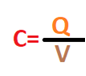C= Capacitance (F)

Q= Electric charge stored in the capacitor

V= Voltage between the plates of a capacitor

Symbolic Representation of a Capacitor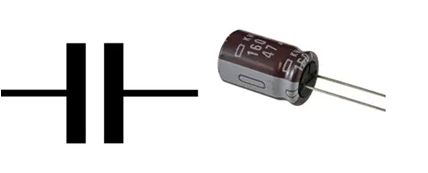Capacitors in Series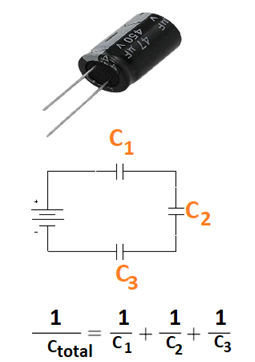Capacitors in Parallel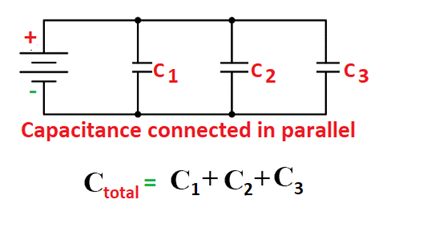Types of Capacitors

• Film Capacitors
• Ceramic Capacitors
• Electrolytic Capacitors
• Variable Capacitors

What is capacitor coupling?

In a process known as Capacitor Coupling, capacitors allow AC to pass through while blocking DC. In the case of a loudspeaker, it is used. Speakers produce sound by converting an alternating current to a direct current, but any direct current that reaches them may cause damage. The direct current is protected from harming the speakers by a capacitor.

What is an Inductor?

An inductor is also called an electrical inductor, it is an electrical component that is designed to store energy in the form of a magnetic field while electric current passes through it. It has two terminals.

The inductor is also known as a coil, chokes, reactor.

The S.I unit of inductance is Henry (H).

The inductance is denoted by the letter “L.”

An iron-core inductor is made up of insulated copper wrapped around an iron core made of plastic or ferromagnetic material.

Inductors are usually available in the 1H (10-6 H) to 20H range.

Symbolic Representation of Inductor

Inductors in Series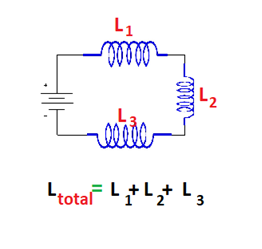Inductors in Parallel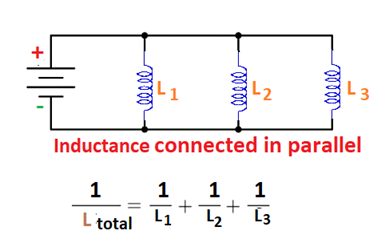Construction of an Inductor

An inductor is formed out of a coil of conducting material (often used as insulated copper wire) wrapped around a core composed of plastic or ferromagnetic material. The use of ferromagnetic core results in a larger inductance.

Low-frequency inductors are often built in the same way that transformers are. They have laminated cores made of electrical steel to assist prevent eddy currents.

Inductors come in a variety of forms and sizes, and some of them include changeable cores that may be adjusted to change the inductance.

Small value inductors are manufactured on integrated circuits using interconnect methods, while planar inductors are made with a planar core. Aluminium interconnects are commonly utilised and fixed in a spiral coil arrangement. Small dimensions, on the other hand, have significant drawbacks. They can resist the inductance.

Shielded inductors are utilised in power regulation systems, lighting, and other systems that require low-noise operation. These inductors are frequently insulated, either partially or completely.

Different Types of Inductors

Different types of inductors are

• Iron Core Inductor
• Air Core Inductor
• Iron Powder Inductor
• Ferrite Core Inductor which is divided into,
• Soft Ferrite
• Hard Ferrite

Capacitor vs Inductor

S.No Capacitor Inductor
1 The capacitor stores electricity in the form of electric charges The inductor stores electricity in the form of magnetic lines
2. Capacitance is represented by the letter C Inductance is represented by the letter L
3. Farad (F) is the unit Henry (H) is the S.I unit of inductance
4. The main role of capacitance is to oppose the change in voltage. The main function of inductance is to oppose the change in electric current.
5. Insulation is provided over the conductor Insulation does not provide over the conductor
6. In capacitor, energy is stored in the form of electrostatic form In inductor, energy is stored in the form of magnetic field
7. Voltage lags current by π/2 Current lags voltage by π/2
8. In a capacitor, the voltage does not change instantly. In an inductor, the current does not change instantly.
9. Used in power supplies Used in home appliance that runs in AC current like TV, Radio, etc.

Application of Capacitor in circuits

• Capacitor used in power supplies
• Capacitor can be used as a sensor to sense air humidity, fuel level etc
• Removing glitches from direct current (DC) power rails

Application of Inductor in circuits

• Inductors are widely utilised in AC applications such as television and radio.
• Chokes - An inductor’s main property is employed in power supply circuits to convert an AC mains supply to a DC supply.
• The energy storage is utilised to provide the spark that ignites the gasoline in car engines.
• Transformers are made up of inductors connected by a distributing magnetic channel.